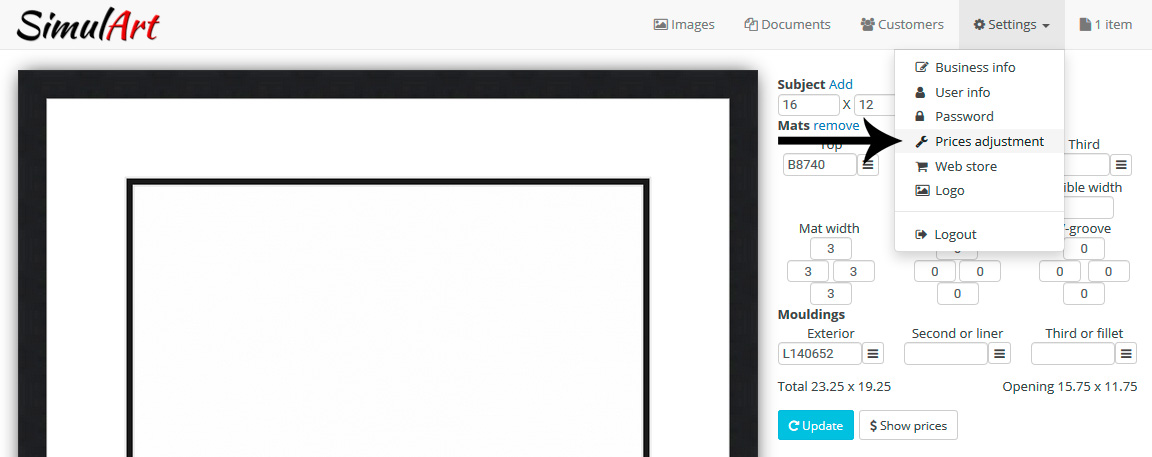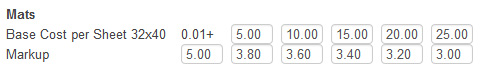### Prices Adjustment (International)

#### Prices calculated by the system may be adjusted by changing the markups or price factors under "Settings". Changes will take effect in both the admin side and online store (if applicable).#### Frame prices are calculated using length costs multiplied by markups. This is the formula used: moulding required (ft or meter) x selected cost (ft or meter) x applicable markup In the example below, a moulding with a cost (ft or meter) between 0.01 and 0.99 will have a markup of 6.00.#### Mat prices are calculated using their base cost per sheet and markups like this: calculated mat size required (sq inch or sq cm) x calculated mat cost based on sheet (sq inch or sq cm) x applicable markup In the example below, a mat with a 32x40 sheet cost between 5.00 and 9.99 will have a markup of 3.80.#### Other components have their own retail prices you can modify, you may also rename some of them. The system will calculate the retail prices under, over, and in between each preset sizes based on half perimeter difference. Items that can be offered in your web store may also be enabled/disabled here as well (if applicable).© SimulArt Inc.